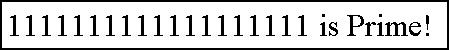The Repunit Primes Project

Theory

 A repunit is a number consisting of copies of the single digit 1. Examples of repunit are 11, 1111, 11111. It's like a repeating unit. A repunit prime is a repunit that is also a prime number. The term "repunit" was coined by A. H. Beiler (1966). Such a number depends on the base that you are using. We concentrate on base-10 in this website. In this base, repunits have the form: Rp=(10^p-1)/9 It is easy to show that if n is divisible by a, then Rn is divisible by Ra. For example, 9 is divisible by 3, and indeed R9 is divisible by R3, in fact, 111111111 = 111 · 1001001. Thus, for Rn to be prime, n must necessarily be prime. But it is not sufficient for n to be prime; for example, R3 = 111 = 3 · 37 is not prime. The factors of repunit numbers Rp, with p prime, are in the form: factor = 2k * p + 1 It's very easy to implement a general algorithm to find factors. The following code is written using a basic-like language: p=43 rp=(10^43-1)/9 for k=1 to 1000000    factor=(2*k*p)+1    remainder=rp % factor    if remainder=0 then print factor next k